';

## Title 40 Part 63 → Subpart HHHHHHH → §63.11950

### Title 40 Part 63 → Subpart HHHHHHH → §63.11950

e-CFR data is current as of August 15, 2019

 Title 40 → Chapter I → Subchapter C → Part 63 → Subpart HHHHHHH → §63.11950

## §63.11950   What emissions calculations must I use for an emission profile?

When developing your emission profiles for batch process vents as required in §63.11925(g), except as specified in paragraph (i) of this section, you must calculate emissions from episodes caused by vapor displacement, purging a partially filled vessel, heating, depressurization, vacuum operations, gas evolution, air drying, or empty vessel purging, using the applicable procedures in paragraphs (a) through (h) of this section.

(a) Vapor displacement. You must calculate emissions from vapor displacement due to transfer of material using Equation 1 of this section.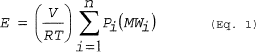View or download PDF

(Eq. 1)

Where:

E = Mass of HAP emitted.

V = Volume of gas displaced from the vessel.

R = Ideal gas law constant.

T = Temperature of the vessel vapor space; absolute.

Pi = Partial pressure of the individual HAP.

MWi = Molecular weight of the individual HAP.

n = Number of HAP compounds in the emission stream.

i = Identifier for a HAP compound.

(b) Gas sweep of a partially filled vessel. You must calculate emissions from purging a partially filled vessel using Equation 2 of this section. The pressure of the vessel vapor space may be set equal to 760 millimeters of mercury (mmHg). You must multiply the HAP partial pressure in Equation 2 of this section by a HAP-specific saturation factor determined in accordance with Equations 3 through 5 of this section. Solve Equation 3 of this section iteratively beginning with saturation factors (in the right-hand side of the equation) of 1.0 for each condensable compound. Stop iterating when the calculated saturation factors for all compounds are the same to two significant figures for subsequent iterations. Note that for multi-component emission streams, saturation factors must be calculated for all condensable compounds, not just the HAP.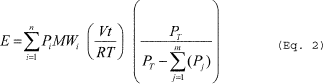View or download PDF

(Eq. 2)

Where:

E = Mass of HAP emitted.

V = Purge flow rate of the noncondensable gas at the temperature and pressure of the vessel vapor space.

R = Ideal gas law constant.

T = Temperature of the vessel vapor space; absolute.

Pi = Partial pressure of the individual HAP at saturated conditions.

Pj = Partial pressure of individual condensable compounds (including HAP) at saturated conditions.

PT = Pressure of the vessel vapor space.

MWi = Molecular weight of the individual HAP.

t = Time of purge.

n = Number of HAP compounds in the emission stream.

i = Identifier for a HAP compound.

j = Identifier for a condensable compound.

m = Number of condensable compounds (including HAP) in the emission stream.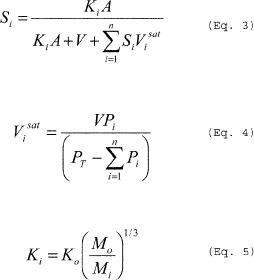View or download PDF

Where:

Si = Saturation factor for individual condensable compounds.

Pi = Partial pressure of individual condensable compounds at saturated conditions.

PT = Pressure of the vessel vapor space.

A = Surface area of liquid.

V = Purge flow rate of the noncondensable gas.

Visat = Volumetric flow rate of individual condensable compounds at saturated vapor pressure.

Ki = Mass transfer coefficient of individual condensable compounds in the emission stream.

Ko = Mass transfer coefficient of reference compound (e.g., 0.83 cm/s for water).

Mo = Molecular weight of reference compound (e.g., 18.02 for water).

Mi = Molecular weight of individual condensable compounds in the emission stream.

n = Number of condensable compounds in the emission stream.

(c) Heating. You must calculate emissions caused by the heating of a vessel to a temperature lower than the boiling point using the procedures in paragraph (c)(1) of this section. If the contents of a vessel are heated to the boiling point, you must calculate emissions using the procedures in paragraph (c)(2) of this section.

(1) If the final temperature to which the vessel contents are heated is lower than the boiling point of the HAP in the vessel, you must calculate the mass of HAP emitted per episode using Equation 6 of this section. The average gas space molar volume during the heating process is calculated using Equation 7 of this section. The difference in the number of moles of condensable in the vessel headspace between the initial and final temperatures is calculated using Equation 8 of this section.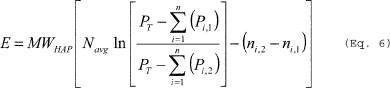View or download PDF

(Eq. 6)

Where:

E = Mass of HAP vapor displaced from the vessel being heated.

Navg = Average gas space molar volume during the heating process.

PT = Total pressure in the vessel.

Pi,1 = Partial pressure of the individual HAP compounds at initial temperature (T1).

Pi,2 = Partial pressure of the individual HAP compounds at final temperature (T2).

MWHAP = Average molecular weight of the HAP compounds calculated using Equation 13 of this section.

ni,1 = Number of moles of condensable in the vessel headspace at initial temperature (T1).

ni,2 = Number of moles of condensable in the vessel headspace at final temperature (T2).

n = Number of HAP compounds in the emission stream.

ln = Natural logarithm.View or download PDF

(Eq. 7)

Where:

Navg = Average gas space molar volume during the heating process.

V = Volume of free space in vessel.

PT = Total pressure in the vessel.

R = Ideal gas law constant.

T1 = Initial temperature of the vessel.

T2 = Final temperature of the vessel.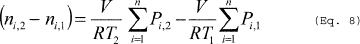View or download PDF

Where:

V = Volume of free space in vessel.

R = Ideal gas law constant.

T1 = Initial temperature in the vessel.

T2 = Final temperature in the vessel.

Pi,1 = Partial pressure of the individual HAP compounds at T1.

Pi,2 = Partial pressure of the individual HAP compounds at T2.

n = Number of HAP compounds in the emission stream.

(2) If the final temperature to which the vessel contents are heated is at the boiling point or higher, you must calculate emissions using the procedure in paragraphs (c)(2)(i) and (ii) of this section.

(i) To calculate the emissions from heating to the boiling point use Equations 9, 10 and 11 of this section. (Note that Pa2 = 0 in the calculation of Δη in Equation 10 of this section.)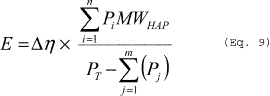View or download PDF

Where:

E = Mass of HAP emitted.

Δη = The number of moles of noncondensable displaced from the vessel, as calculated using Equation 10 of this section.

PT = Pressure in the receiver.

Pi = Partial pressure of the individual HAP determined at the exit temperature of the condenser or at the conditions of the dedicated receiver.

Pj = Partial pressure of the individual condensable (including HAP) determined at the exit temperature of the condenser or at the conditions of the dedicated receiver.

n = Number of HAP compounds in the emission stream.

i = Identifier for a HAP compound.

j = Identifier for a condensable compound.

MWHAP = The average molecular weight of HAP in vapor exiting the dedicated receiver, as calculated using Equation 11 of this section with partial pressures determined at the exit temperature and exit pressure conditions of the condenser or at the conditions of the dedicated receiver.

m = Number of condensable compounds (including HAP) in the emission stream.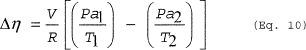View or download PDF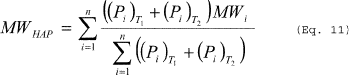View or download PDF

Where:

Δη = Number of moles of noncondensable gas displaced from the vessel.

V = Volume of free space in the vessel.

R = Ideal gas law constant.

T1 = Initial temperature of vessel contents, absolute.

T2 = Final temperature of vessel contents, absolute.

Pan = Partial pressure of noncondensable gas in the vessel headspace at initial (n = 1) and final (n = 2) temperature.

MWHAP = The average molecular weight of HAP in vapor exiting the dedicated receiver.

(Pi)Tn = Partial pressure of each HAP in the vessel headspace at initial (T1) and final (T2) temperature of the receiver.

MWi = Molecular weight of the individual HAP.

n = Number of HAP compounds in the emission stream.

i = Identifier for a HAP compound.

(ii) While boiling, the vessel must be operated with a properly operated process condenser. An initial demonstration that a process condenser is properly operated must be conducted during the boiling operation and documented in the notification of compliance status report described in §63.11985(a). You must either measure the liquid temperature in the receiver or the temperature of the gas stream exiting the condenser and show it is less than the boiling or bubble point of the HAP in the vessel; or perform a material balance around the vessel and condenser and show that at least 99 percent of the recovered HAP vaporized while boiling is condensed. This demonstration is not required if the process condenser is followed by a condenser acting as a control device or if the control device is monitored using a CEMS.

(d) Depressurization. You must calculate emissions from depressurization using Equation 12 of this section.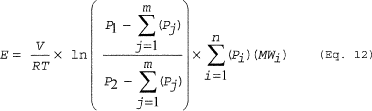View or download PDF

Where:

E = Emissions.

V = Free volume in vessel being depressurized.

R = Ideal gas law constant.

T = Temperature of the vessel, absolute.

P1 = Initial pressure in the vessel.

P2 = Final pressure in the vessel.

Pj = Partial pressure of the individual condensable compounds (including HAP).

MWi = Molecular weight of the individual HAP compounds.

n = Number of HAP compounds in the emission stream.

m = Number of condensable compounds (including HAP) in the emission stream.

i = Identifier for a HAP compound.

j = Identifier for a condensable compound.

ln = Natural logarithm.

(e) Vacuum systems. You must calculate emissions from vacuum systems using Equation 13 of this section if the air leakage rate is known or can be approximated. The receiving vessel is part of the vacuum system for purposes of this subpart.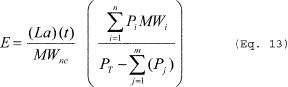View or download PDF

Where:

E = Mass of HAP emitted.

PT = Absolute pressure of receiving vessel or ejector outlet conditions, if there is no receiver.

Pi = Partial pressure of the HAP at the receiver temperature or the ejector outlet conditions.

Pj = Partial pressure of condensable (including HAP) at the receiver temperature or the ejector outlet conditions.

La = Total air leak rate in the system, mass/time.

MWnc = Molecular weight of noncondensable gas.

t = Time of vacuum operation.

MWi = Molecular weight of the individual HAP in the emission stream, with HAP partial pressures calculated at the temperature of the receiver or ejector outlet, as appropriate.

(f) Gas evolution. You must calculate emissions from gas evolution using Equation 13 in paragraph (e) of this section with mass flow rate of gas evolution, Wg, substituted for La.

(g) Air drying. You must calculate emissions from air drying using Equation 14 of this section: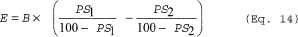View or download PDF

Where:

E = Mass of HAP emitted.

B = Mass of dry solids.

PS1 = HAP in material entering dryer, weight percent.

PS2 = HAP in material exiting dryer, weight percent.

(h) Empty vessel purging. You must calculate emissions from empty vessel purging using Equation 15 of this section (Note: The term e-Ft/v can be assumed to be 0):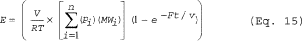View or download PDF

Where:

V = Volume of empty vessel.

R = Ideal gas law constant.

T = Temperature of the vessel vapor space; absolute.

Pi = Partial pressure of the individual HAP at the beginning of the purge.

MWi = Molecular weight of the individual HAP.

F = Flow rate of the purge gas.

t = Duration of the purge.

n = Number of HAP compounds in the emission stream.

i = Identifier for a HAP compound.

(i) Engineering assessments. You must conduct an engineering assessment to calculate HAP emissions or emission episodes from each process vent that are not due to vapor displacement, partially filled vessel purging, heating, depressurization, vacuum operations, gas evolution, air drying or empty vessel purging. An engineering assessment may also be used to support a finding that the emissions estimation equations in this section are inappropriate. All data, assumptions and procedures used in the engineering assessment must be documented, are subject to preapproval by the Administrator, and must be reported in the batch precompliance report. An engineering assessment should include, but is not limited to, the items listed in paragraphs (i)(1) through (4) of this section.

(1) Previous test results provided the tests are representative of current operating practices at the process unit.

(2) Bench-scale or pilot-scale test data representative of the process under representative operating conditions.

(3) Maximum flow rate, HAP emission rate, concentration, or other relevant parameter specified or implied within a permit limit applicable to the process vent.

(4) Design analysis based on accepted chemical engineering principles, measurable process parameters, or physical or chemical laws or properties. Examples of analytical methods include, but are not limited to the following:

(i) Use of material balances based on process stoichiometry to estimate maximum organic HAP concentrations.

(ii) Estimation of maximum flow rate based on physical equipment design such as pump or blower capacities.

(iii) Estimation of HAP concentrations based on saturation conditions.